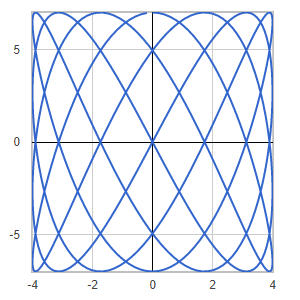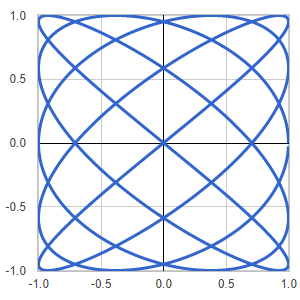### Free Ginckers

#### Lissajous Curve (3, 2)

Template: Graphics/2d-parametric-chart

Discription: In mathematics, a Lissajous curve is a graph of a system of parametric equations that describe complex harmonic motion. The appearance of the curve is highly sensitive to the ratio of a/b .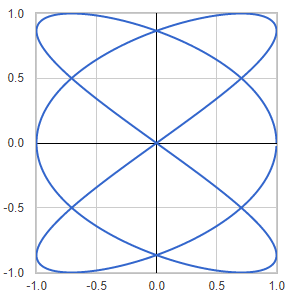#### Archemedean Spiral

Template: Graphics/2d-parametric-chart

Discription: The Archemedean spiral is a spiral named after Greek mathematician Archimedes. It is the locus of points corresponding to the locations over time of a point moving away from a fixed point with a constant speed along a line rotates with constant angular velocity.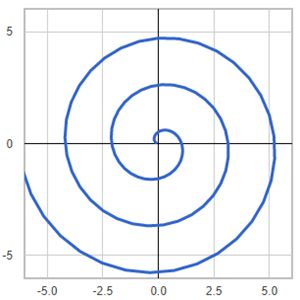#### Bean

Template: Graphics/2d-parametric-chart

Discription: Bean shape created using 2D parametric equations.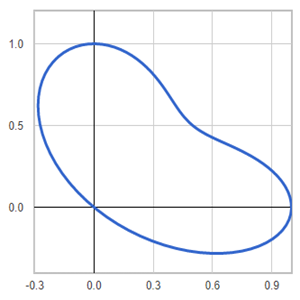#### Butterfly

Template: Graphics/2d-parametric-chart

Discription: Butterfly shape created using 2D parametric equations.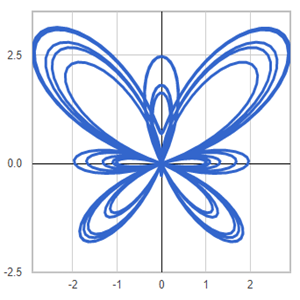#### Circles

Template: Graphics/2d-parametric-chart

Discription: Circles created using 2D parametric equations.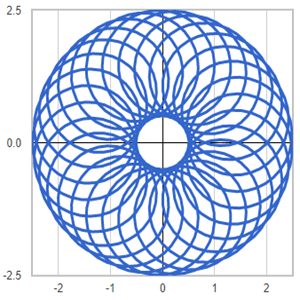#### Circular

Template: Graphics/2d-parametric-chart

Discription: Circular shape created using 2D parametric equations.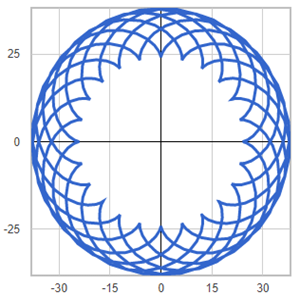#### Cycloids (1, 3)

Template: Graphics/2d-parametric-chart

Discription: Cycloid is the trace of a point on a circle rolling upon another circle without slipping. When a circle is rolling externally upon a fixed circle - in the same manner a coin rolls around another - we have epicycloid. When the rolling is internal, we have hypocycloid.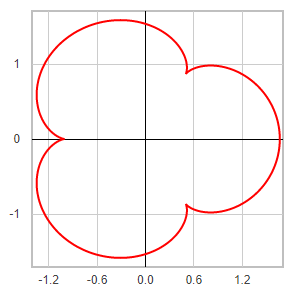#### Cycloids (1, -6)

Template: Graphics/2d-parametric-chart

Discription: Cycloid is the trace of a point on a circle rolling upon another circle without slipping. When a circle is rolling externally upon a fixed circle - in the same manner a coin rolls around another - we have epicycloid. When the rolling is internal, we have hypocycloid.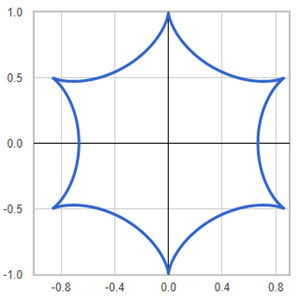#### Cycloids (2, -7)

Template: Graphics/2d-parametric-chart

Discription: Cycloid is the trace of a point on a circle rolling upon another circle without slipping. When a circle is rolling externally upon a fixed circle - in the same manner a coin rolls around another - we have epicycloid. When the rolling is internal, we have hypocycloid.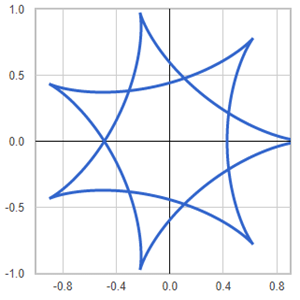#### Cycloids (3, -7)

Template: Graphics/2d-parametric-chart

Discription: Cycloid is the trace of a point on a circle rolling upon another circle without slipping. When a circle is rolling externally upon a fixed circle - in the same manner a coin rolls around another - we have epicycloid. When the rolling is internal, we have hypocycloid.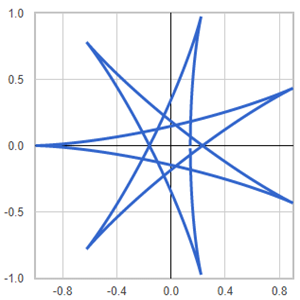#### Cycloids (5, 1)

Template: Graphics/2d-parametric-chart

Discription: Cycloid is the trace of a point on a circle rolling upon another circle without slipping. When a circle is rolling externally upon a fixed circle - in the same manner a coin rolls around another - we have epicycloid. When the rolling is internal, we have hypocycloid.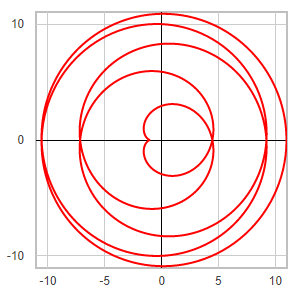#### Cycloids (6, 5)

Template: Graphics/2d-parametric-chart

Discription: Cycloid is the trace of a point on a circle rolling upon another circle without slipping. When a circle is rolling externally upon a fixed circle - in the same manner a coin rolls around another - we have epicycloid. When the rolling is internal, we have hypocycloid.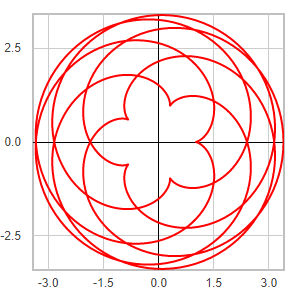#### Cycloids (8, -21)

Template: Graphics/2d-parametric-chart

Discription: Cycloid is the trace of a point on a circle rolling upon another circle without slipping. When a circle is rolling externally upon a fixed circle - in the same manner a coin rolls around another - we have epicycloid. When the rolling is internal, we have hypocycloid.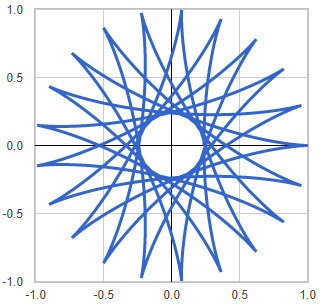#### Flower

Template: Graphics/2d-parametric-chart

Discription: Flower shape created using 2D parametric equations.#### Lattice(16, 19)

Template: Graphics/2d-parametric-chart

Discription: Lattice shape created using 2D parametric equations.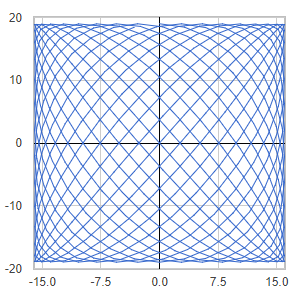#### Lattice(2, 3)

Template: Graphics/2d-parametric-chart

Discription: Lattice shape created using 2D parametric equations.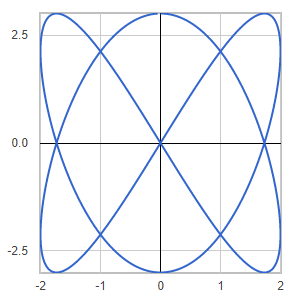#### Lattice(4, 7)

Template: Graphics/2d-parametric-chart

Discription: Lattice shape created using 2D parametric equations.